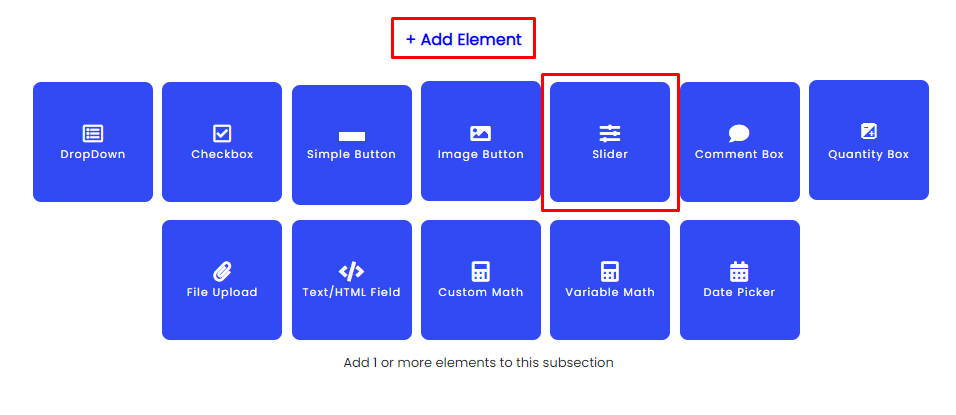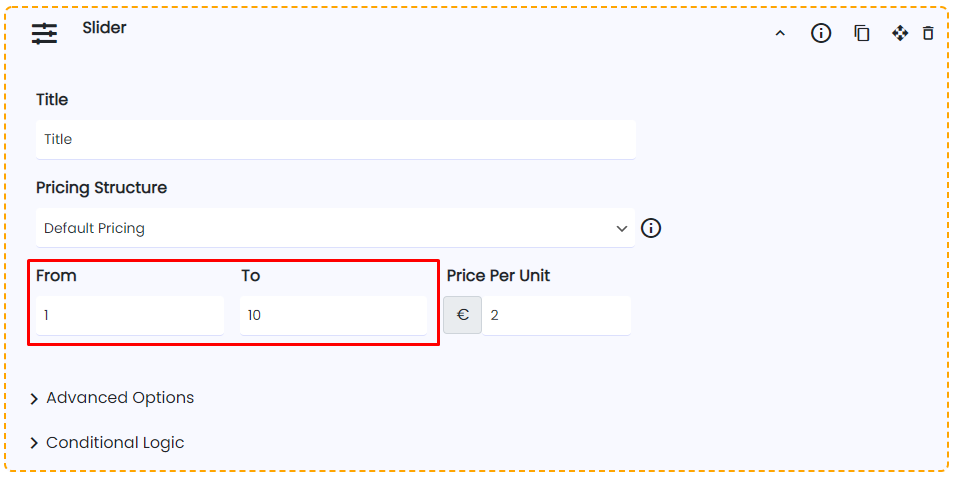Introduction

Sliders are a very common and widely spread UI pattern. It allows users to select a value within a range of numbers that you can manually set.

In the following sections, we will provide you with all the information you need to set up your sliders.

Benefits, Features & Use Cases

Range sliders have a technical advantage over the input field as it will require less validation to be sure. For the same reason, it can benefit the user because a range slider will not generate an unnecessary error due to an “incorrect” entry.

Range sliders work great when you have a strictly defined range that can only have a small number of values. For example, guest meal reservations or maybe a volume control.

Sliders are linked to any elements in the same subsection.
1. It can act as a multiplier of other elements.

2. It can act as a product selector. Buy X amount of item.

Watch It In-ActionHow to Activate & Use This Feature

To add a slider, simply click on the "+ Add Element" button and then "Slider"Settings & Options

The title is an easy one, let's continue with "Pricing Structure" and here is when things get interesting:1. Default Pricing

The standard way of using a slider is you determine how much each unit will cost a product for service.
-> For example, from 1 apple to 10 apples, it's \$1.50 per apple.

2. Bulk Discounts (Quantity Discounts)

- Calculates at a per-unit cost.

Prince ranges:
Between 1 and 5 units = \$50 (per unit price)
Between 6 and 10 units = \$45 (per unit price)
Between 11 and 20 units = \$45 (per unit price)
Prices:
3 Units = \$150 (total price)
8 Units =\$360 (total price)
15 Units= \$675 (total price)

3. Sliding Scale

Set a flat price between price ranges

- Calculates at a total range cost.

Prince ranges:
Between 1 and 10 units = \$100 (flat price)
Between 11 and 20 units = \$200 (flat price)
Between 21 and 30 units = \$300 (flat price)
Prices:
3 Units = \$100 (total price)
15 Units =\$200 (total price)
25 Units= \$300 (total price)

4. Element Quantity Modifier Only

- Used to change the select quantity of an element in the same subsection.

For example, if you have checkboxes asking the users to select the fruits they want to buy, then you have this slider for the user to select how many items they want to buy.

You can set the starting value "From" and the end value "To" to create the sliding interval."Required" by activating this option, users will be forced to select an option before being able to continue to checkout or a price quote."Price Hint" shows the user a temporary price bubble that shows the amount added to the total when they select this item.You can choose if you want this item to be displayed on the Detailed List & PDFWhen activating the "Quantity Input Box" it will add a quantity input box to the end of the slider, linking both elements togetherThe "starting value" will be the starting point of the slider and the "slider steps" are the number of numbers the slider will jump every time you slide it.Finally, the "Tooltip" will create a frontend information icon so you can better explain to the customers what this item is about while keeping your calculator form organized.Video Tutorial

https://youtu.be/HrEC57exom8?t=199